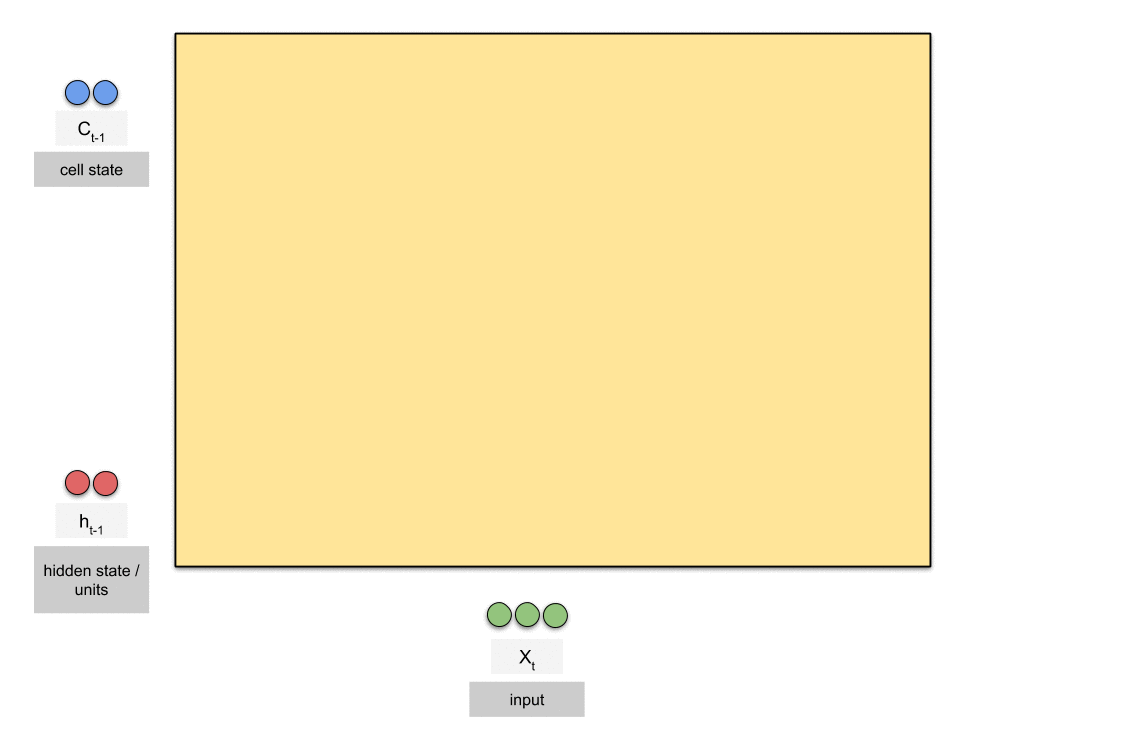# Week 5 — Audio Emotion Recognition System (Part III)

Source: Deep Learning on MediumFirstly, we trained our model by using LSTM. We used 9 LSTM layers and each of them has 50 units, ‘adam’ as optimizer, ‘sparse categorical cross entropy’ as loss function, epoch 100, return sequence=true, softmax as activation function in last layer. In dense layer, we used 9 as units parameter because we have 8 different labels. Average result is 0,35. (mfcc feature as input)

Secondly, we created CNN models with both sound amplitude images and mfcc features as input. The average result of CNN model with mfcc is 0.67. We used 4 Conv1D layers, 4 activation layers with ReLU as activation function, 1 Max pooling layer, 1 flatten layer, 1 dense layer and softmax activation function in last layer. Learning rate is 0.00001 and epoch is 100.

Then, we created CNN models with sound amplitude images as input. The average result of CNN model with images is 0.35. We used 3 Conv2D layers, 2 Max pooling layer, 1 flatten layer, 1 dense layer and softmax activation function in last layer. Epoch is 50.

Then, we used Random Forest, Gradient Boost, Catboost as classifier. In order to use these machine learning methods, we took mean values of “mfcc”, “chroma_stft”, “chroma_cqt”, “chroma_cens”, “rms”, “spectral_contrast”, “spectral_bandwidth”, “tonnetz”, “zcr”. Our accuracy results are:

Random forest : 0.43